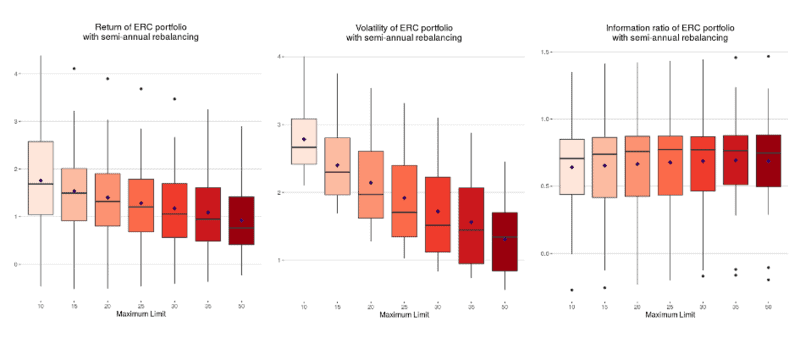# How A Maximum Weight Limit Affects the Returns of ERC Portfolios

Adding a maximum weight limit, or cap, to an equal risk contribution (ERC) portfolio does not affect its information ratio – though lower weight caps are associated with higher return and volatility.

The information ratio measures excess returns per unit of volatility risk, essentially gauging how consistent a strategy’s outperformance over a benchmark is. The higher the information ratio, the better.

A study by Quantilia found that maximum weight caps do not tend to affect the information ratios of ERC-weighted portfolios or inverse-volatility portfolios. In both cases, however, lower weight caps tended to generate higher volatility and higher mean rolling returns.This is because under ERC and inverse-volatility weighting schemes, a few strategies often make up most of the total portfolio’s weight. By restricting the weightings of these strategies, more weight is shifted to strategies that contribute higher volatility and risk – leading to higher portfolio volatility and returns.

ERC funds are weighted in such a way that each security contributes equally to total portfolio risk, thereby reducing the risk concentration of the portfolio. This is similar to the inverse-volatility approach, though the ERC strategy also accounts for the correlations between securities within the portfolio.

The Quantilia study used a range of weighting caps, from 10% to 50%. The report was based on 20 baskets of 20 strategies each, with a split between alpha and beta strategies and exposure to different asset classes and strategy providers. The study assumed transaction costs of 20 basis points and used a semi-annual rebalancing strategy.

It also found that implementing lower weight limits, or weight floors, affected these portfolios’ return, volatility and information ratio figures. If weight floors are raised, information ratios tend to decrease. The study suggests using lower weight limits of only 0.5% or 1%.